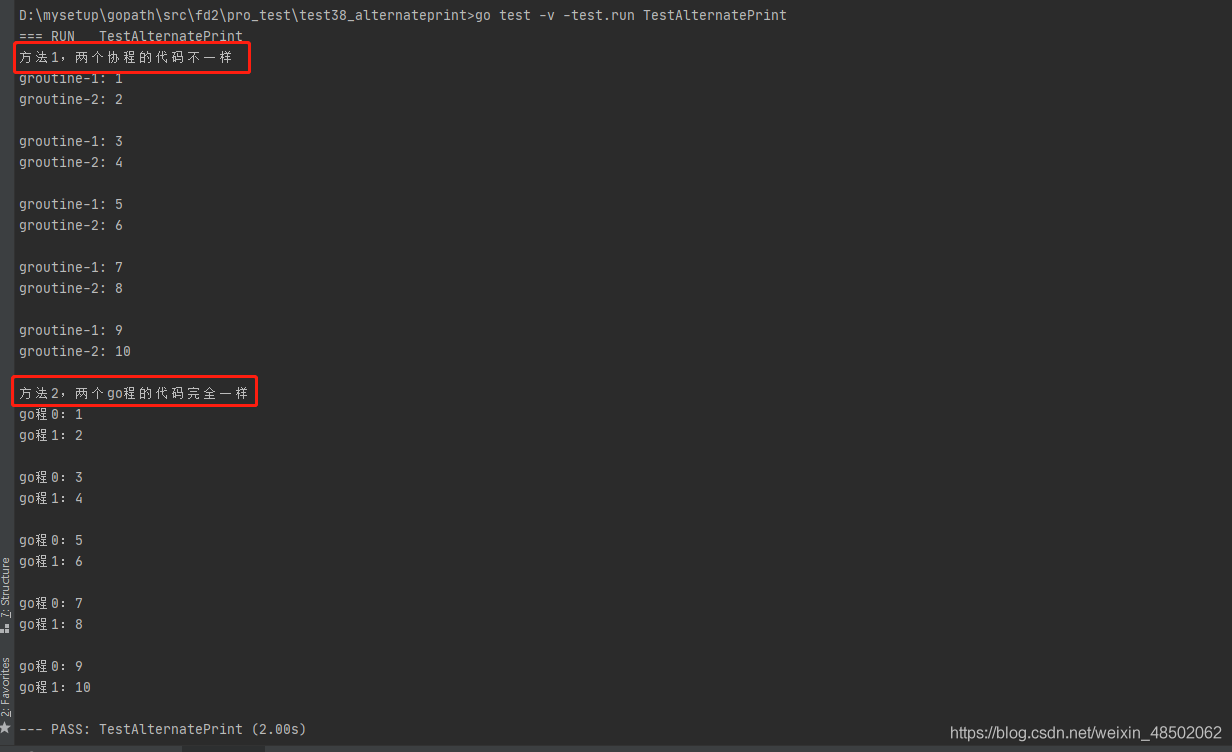# September 9, 2020: naked writing algorithm: two threads print numbers 1-100 in turn.

2020-11-06 21:50:36

Method 1： With a channel , Two go The program code is different .
Method 2： Two channels , Two go The program code is exactly the same . It can be extended to N individual go Cheng takes turns printing .

The code to use golang To write , The code is as follows ：

``````package test38_alternateprint

import (
"fmt"
"testing"
"time"
)

var POOL = 10

//go test -v -test.run TestAlternatePrint
func TestAlternatePrint(t *testing.T) {
AlternatePrint1()
AlternatePrint2()
}

// Method 1
func AlternatePrint1() {
fmt.Println(" Method 1, The codes of the two coroutines are different ")
msg := make(chan int)
go func(p chan int) {
for i := 1; i <= POOL; i++ {
p <- i
if i%2 == 1 {
fmt.Println("groutine-1:", i)
}
}
}(msg)
go func(p chan int) {
for i := 1; i <= POOL; i++ {
<-p
if i%2 == 0 {
fmt.Println("groutine-2:", i)
fmt.Println("")
}
}
}(msg)

// Wait for the execution of the cooperation process to complete
time.Sleep(time.Second * 1)
}

// Method 2
func AlternatePrint2() {
fmt.Println(" Method 2, Two go The program code is exactly the same ")
const N = 2
chs := make([]chan struct{}, N)
for i := 0; i < N; i++ {
chs[i] = make(chan struct{}, 0)
}
start := 1
for i := 0; i < N; i++ {
go func(i int) {
for start <= POOL-N+1 {
// Get executive power
<-chs[i]

// Execute code
fmt.Printf("go cheng %d：%d\r\n", i, start)
if (i+1)%N == 0 {
fmt.Println("")
}
start++

// Give other programs the right to execute
chs[(i+1)%N] <- struct{}{}
}
}(i)
}

// Give it to 1 The right to execute a contract , The first 1 The sequence number of the coroutines is 0
chs <- struct{}{}

// Wait for the execution of the cooperation process to complete
time.Sleep(time.Second * 1)

// Take back the last 1 individual go The executive power of Cheng
<-chs[POOL%N]
}``````

knock go test -v -test.run TestAlternatePrint command , give the result as follows ：Comment on